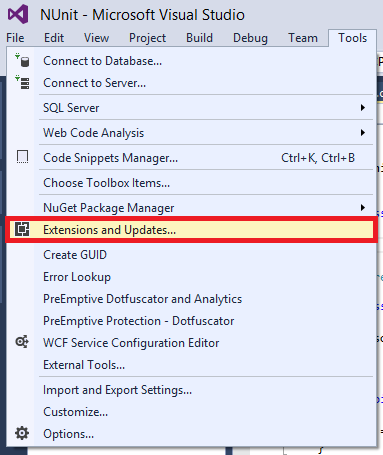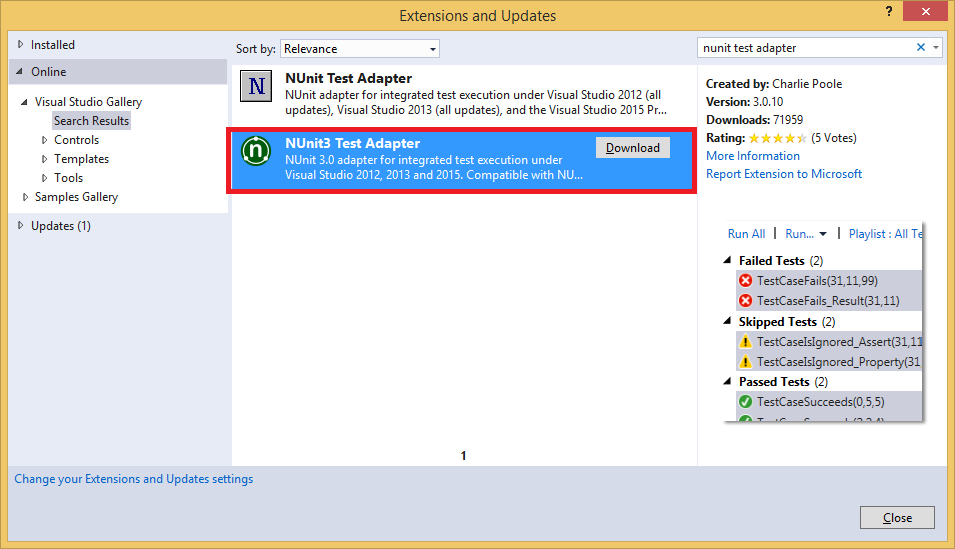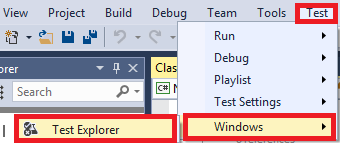Start new console application. In the solution add new class library. Then, in that class library add reference to NUnit, in my case file is:

C:\Program Files (x86)\NUnit.org\framework\3.2.1.0\net-4.5\nunit.framework.dll

Also add reference in class library to console application (application which we are going to test)

We will also need NUnit Test Adapter, in order to see our tests in test explorer.

Go to Tools -> Extensions and UpdatesGo to online and search for NUnit3 Test Adapter - in my case I needed NUnit3 because of VS 2015Install it. Restart VS. Code in console application which I am going to test looks like:

```namespace NUnit
{
public class Program
{
static void Main(string[] args)
{
}

public string ATest()
{
return "Hello World";
}
}
}
```

Class library looks like:

```using NUnit.Framework;
using NUnit;

namespace NUnitTest
{
[TestFixture]
public class HelloWorldTest
{
Program source;

[SetUp]
public void Init()
{
source = new Program();
}

[Test]
public void ShouldShowHelloWorld()
{
Assert.AreEqual("Hello World", source.ATest());
}
}
}
```

Now rebuild solution. Open Test Explorer (Test -> Window -> Test Explorer)And run all tests:For parameterized tests I've created solution with two projects, one is console application, and second one (where my tests are actually) is class library.

My main, console, application looks like this:

```static void Main(string[] args)
{
Summation mySum = new Summation();
Console.WriteLine(mySum.MySummation(10).ToString());
}
```

Where class "Summation" is like:

```public class Summation
{
public int MySummation(int myValue)
{
return myValue + myValue;
}
}
```

Now, let's say that we want to create unit test for 10, 20 and 30 values. Instead of writing three tests we will use parameterized tests like:

```[Test]
[Sequential]
public void Summ_results([Values(10, 20, 30)] int sumResult)
{
Summation mySum = new Summation();
Assert.AreEqual(sumResult + sumResult, mySum.MySummation(sumResult));
}
```

Notice part:

[Values(10, 20, 30)]

With that line and class attribute [Sequential] we said that our test will be executed three times where each time sumResult will be 10, 20 or 30.